# Examples¶

BurnMan comes with a large collection of example programs under examples/. Below you can find a summary of the different examples. They are grouped into Simple Examples and More Advanced Examples. We suggest starting with the Tutorial before moving on to the examples, especially if you are new to using BurnMan.

Finally, we also include the scripts that were used for all computations and figures in the 2014 BurnMan paper in the misc/ folder, see Reproducing Cottaar, Heister, Rose and Unterborn (2014).

## Simple Examples¶

The following is a list of simple examples:

### example_beginner¶

This example script is intended for absolute beginners to BurnMan. We cover importing BurnMan modules, creating a composite material, and calculating its seismic properties at lower mantle pressures and temperatures. Afterwards, we plot it against a 1D seismic model for visual comparison.

Uses:

Demonstrates:

• creating basic composites
• calculating thermoelastic properties
• seismic comparison

Resulting figure: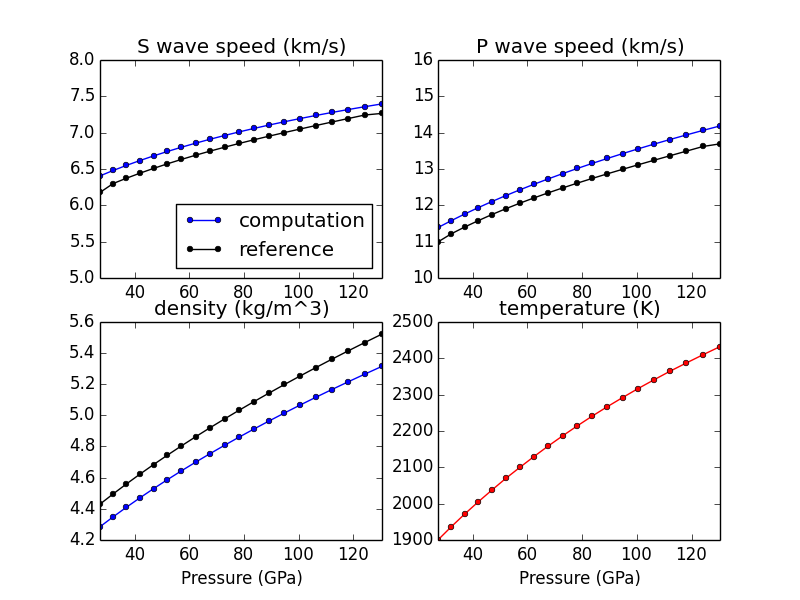### example_solid_solution¶

This example shows how to create different solid solution models and output thermodynamic and thermoelastic quantities.

There are four main types of solid solution currently implemented in BurnMan:

1. Ideal solid solutions
2. Symmmetric solid solutions
3. Asymmetric solid solutions
4. Subregular solid solutions

These solid solutions can potentially deal with:

• Disordered endmembers (more than one element on a crystallographic site)
• Site vacancies
• More than one valence/spin state of the same element on a site

Uses:

Demonstrates:

• Different ways to define a solid solution
• How to set composition and state
• How to output thermodynamic and thermoelastic properties

Resulting figures:### example_geotherms¶

This example shows each of the geotherms currently possible with BurnMan. These are:

1. Brown and Shankland, 1981 [BS81]
2. Anderson, 1982 [And82]
3. Watson and Baxter, 2007 [WB07]
4. linear extrapolation
5. Read in from file from user
6. Adiabatic from potential temperature and choice of mineral

Uses:

Demonstrates:

• the available geotherms

Resulting figure: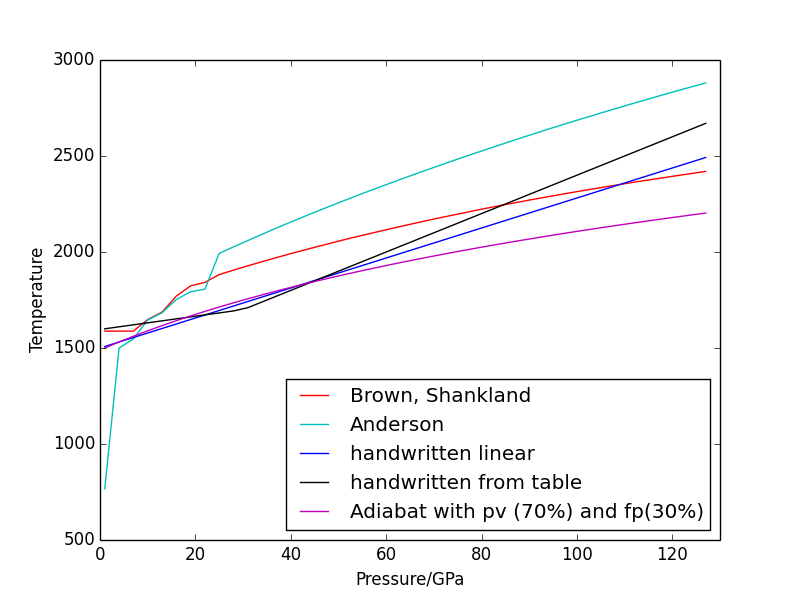### example_seismic¶

Shows the various ways to input seismic models ($$V_s, V_p, V_{\phi}, \rho$$) as a function of depth (or pressure) as well as different velocity model libraries available within Burnman:

1. PREM [DA81]
2. STW105 [KED08]
3. AK135 [KEB95]
4. IASP91 [KE91]

This example will first calculate or read in a seismic model and plot the model along the defined pressure range. The example also illustrates how to import a seismic model of your choice, here shown by importing AK135 [KEB95].

Uses:

Demonstrates:

• Utilization of library seismic models within BurnMan
• Input of user-defined seismic models

Resulting figures: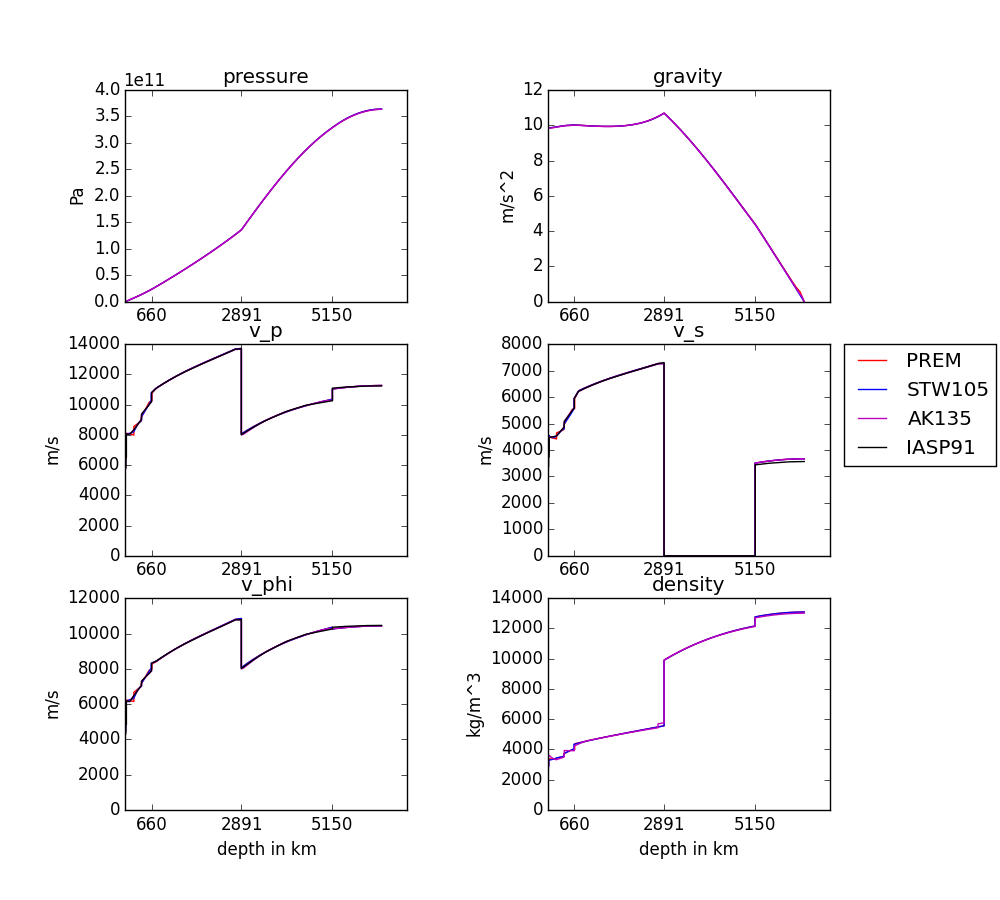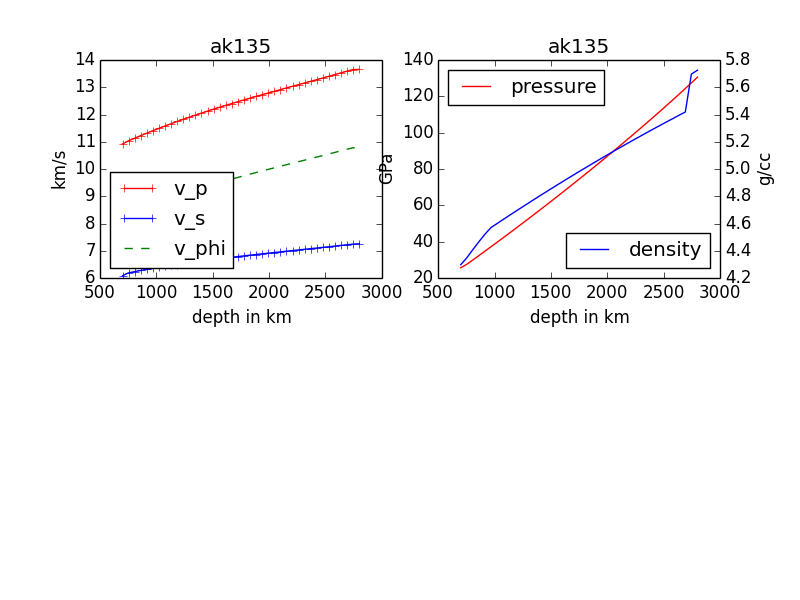### example_composition¶

This example shows how to create different minerals, how to compute seismic velocities, and how to compare them to a seismic reference model.

There are many different ways in BurnMan to combine minerals into a composition. Here we present a couple of examples:

1. Two minerals mixed in simple mole fractions. Can be chosen from the BurnMan libraries or from user defined minerals (see example_user_input_material)
2. Example with three minerals
3. Using preset solid solutions
4. Defining your own solid solution

To turn a method of mineral creation “on” the first if statement above the method must be set to True, with all others set to False.

Note: These minerals can include a spin transition in (Mg,Fe)O, see example_spintransition.py for explanation of how to implement this

Uses:

Demonstrates:

• Different ways to define a composite
• Using minerals and solid solutions
• Compare computations to seismic models

Resulting figure:### example_averaging¶

This example shows the effect of different averaging schemes. Currently four averaging schemes are available:

1. Voight-Reuss-Hill
2. Voight averaging
3. Reuss averaging
4. Hashin-Shtrikman averaging

See [WDOConnell76] Journal of Geophysics and Space Physics for explanations of each averaging scheme.

Specifically uses:

Demonstrates:

• implemented averaging schemes

Resulting figure: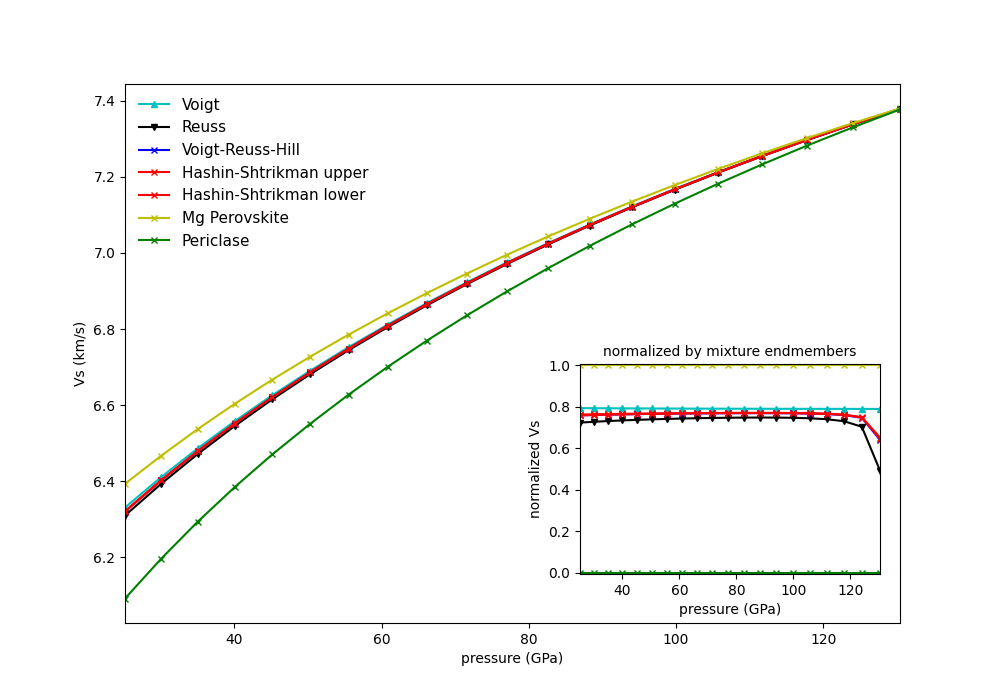### example_chemical_potentials¶

This example shows how to use the chemical potentials library of functions.

Demonstrates:

• How to calculate chemical potentials
• How to compute fugacities and relative fugacities

Resulting figure:### example_spintransition¶

This example shows the different minerals that are implemented with a spin transition. Minerals with spin transition are implemented by defining two separate minerals (one for the low and one for the high spin state). Then a third dynamic mineral is created that switches between the two previously defined minerals by comparing the current pressure to the transition pressure.

Specifically uses:

Demonstrates:

• implementation of spin transition in (Mg,Fe)O at user defined pressure

Resulting figure: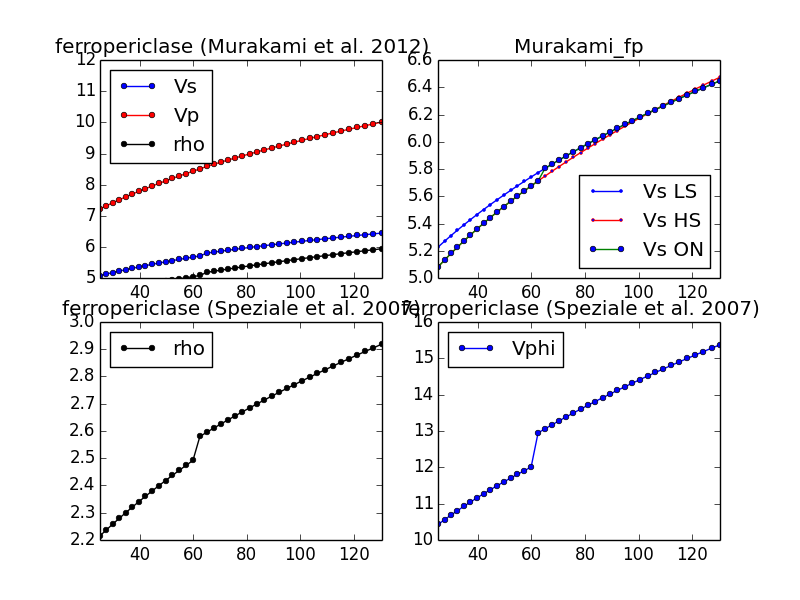### example_user_input_material¶

Shows user how to input a mineral of his/her choice without usint the library and which physical values need to be input for BurnMan to calculate $$V_P, V_\Phi, V_S$$ and density at depth.

Specifically uses:

Demonstrates:

• how to create your own minerals

### example_optimize_pv¶

Vary the amount perovskite vs. ferropericlase and compute the error in the seismic data against PREM. For more extensive comments on this setup, see tutorial/step_2.py

Uses:

Demonstrates:

• compare errors between models
• loops over models

Resulting figure: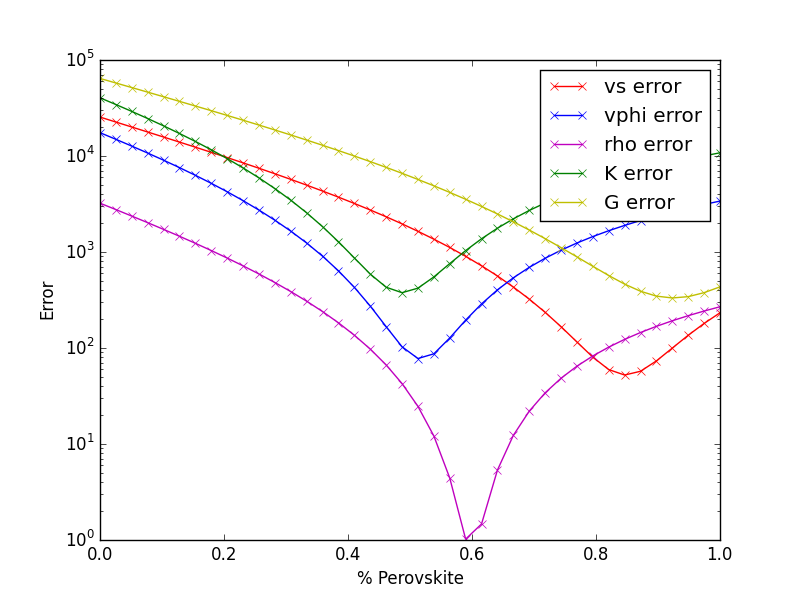### example_build_planet¶

For Earth we have well-constrained one-dimensional density models. This allows us to calculate pressure as a funcion of depth. Furthermore, petrologic data and assumptions regarding the convective state of the planet allow us to estimate the temperature.

For planets other than Earth we have much less information, and in particular we know almost nothing about the pressure and temperature in the interior. Instead, we tend to have measurements of things like mass, radius, and moment-of-inertia. We would like to be able to make a model of the planet’s interior that is consistent with those measurements.

However, there is a difficulty with this. In order to know the density of the planetary material, we need to know the pressure and temperature. In order to know the pressure, we need to know the gravity profile. And in order to the the gravity profile, we need to know the density. This is a nonlinear problem which requires us to iterate to find a self-consistent solution.

Here we show an example that does this, using the planet Mercury as motivation.

Uses:

Resulting figure: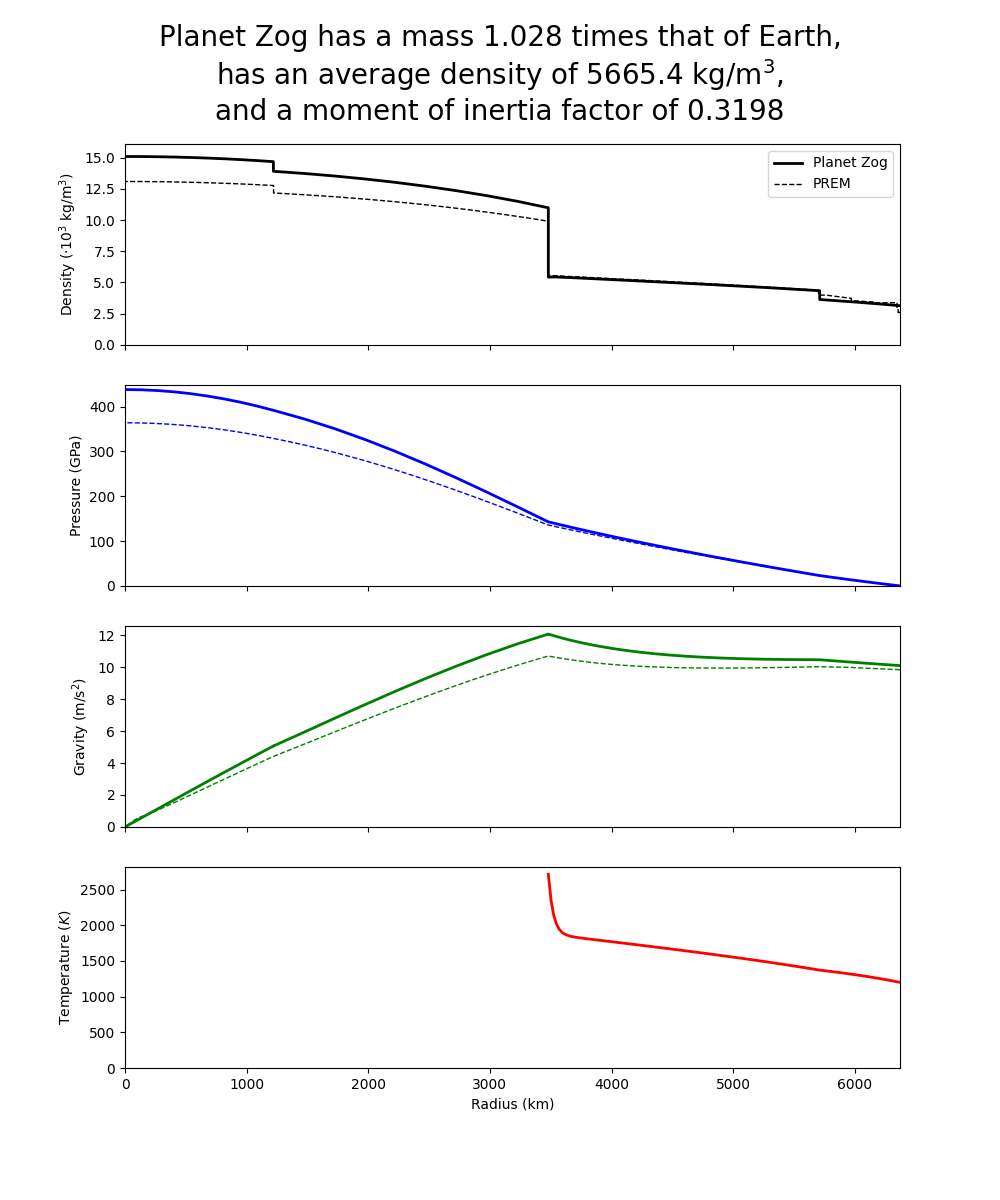### example_compare_all_methods¶

This example demonstrates how to call each of the individual calculation methodologies that exist within BurnMan. See below for current options. This example calculates seismic velocity profiles for the same set of minerals and a plot of $$V_s, V_\phi$$ and $$\rho$$ is produce for the user to compare each of the different methods.

Specifically uses:

Demonstrates:

• Each method for calculating velocity profiles currently included within BurnMan

Resulting figure: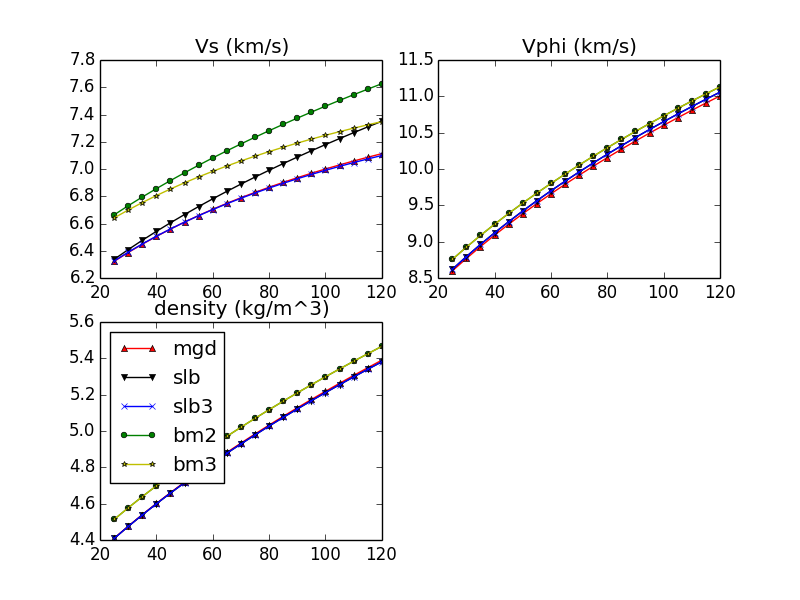### example_anisotropy¶

This example illustrates the basic functions required to convert an elastic stiffness tensor into elastic properties.

Specifically uses:

• burnman.AnisotropicMaterial

Demonstrates:

• anisotropic functions

Resulting figure: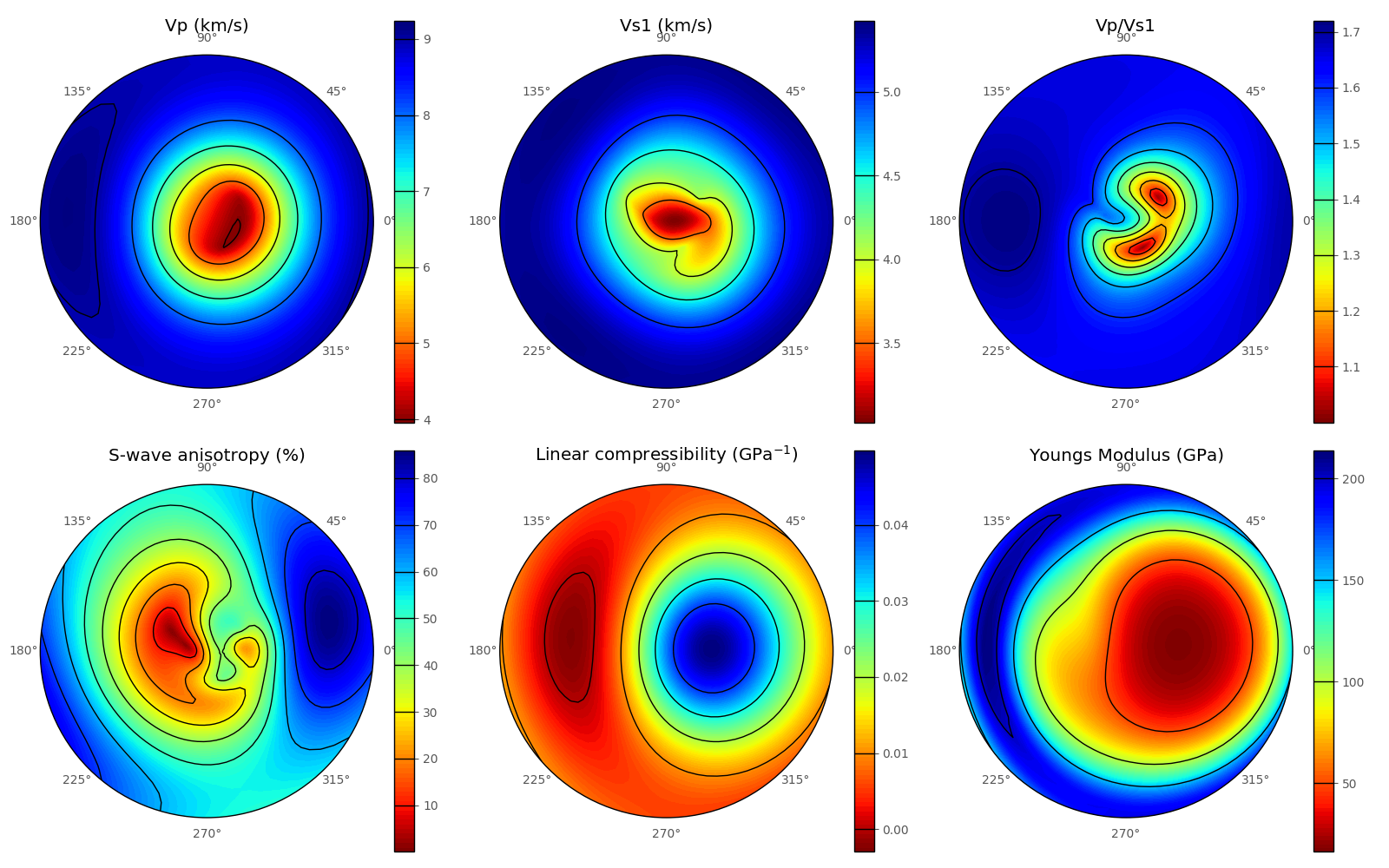### example_fit_data¶

This example demonstrates BurnMan’s functionality to fit various mineral physics data to an EoS of the user’s choice.

Please note also the separate file example_fit_eos.py, which can be viewed as a more advanced example in the same general field.

teaches: - least squares fitting

Resulting figures: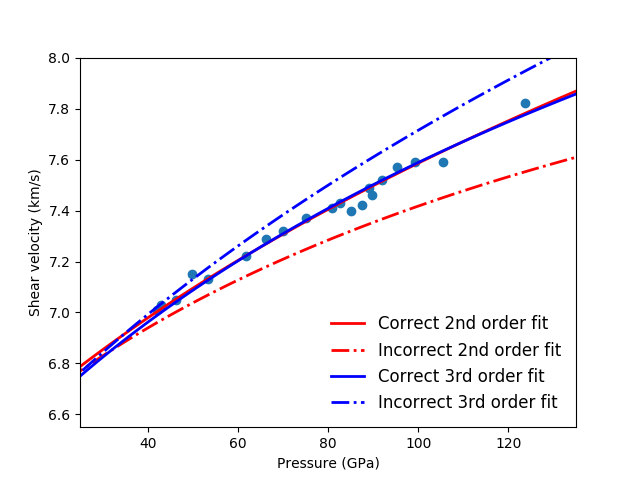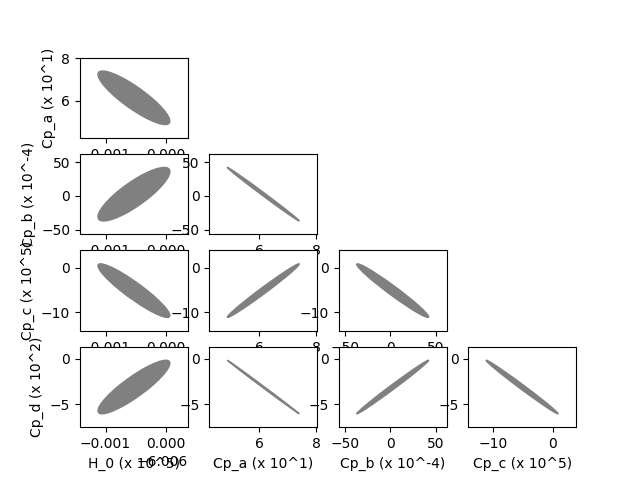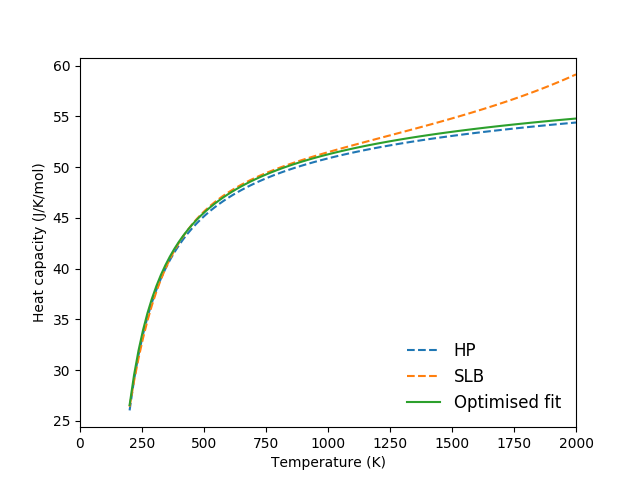### example_fit_eos¶

This example demonstrates BurnMan’s functionality to fit data to an EoS of the user’s choice.

The first example deals with simple PVT fitting. The second example illustrates how powerful it can be to provide non-PVT constraints to the same fitting problem.

teaches: - least squares fitting

Last seven resulting figures: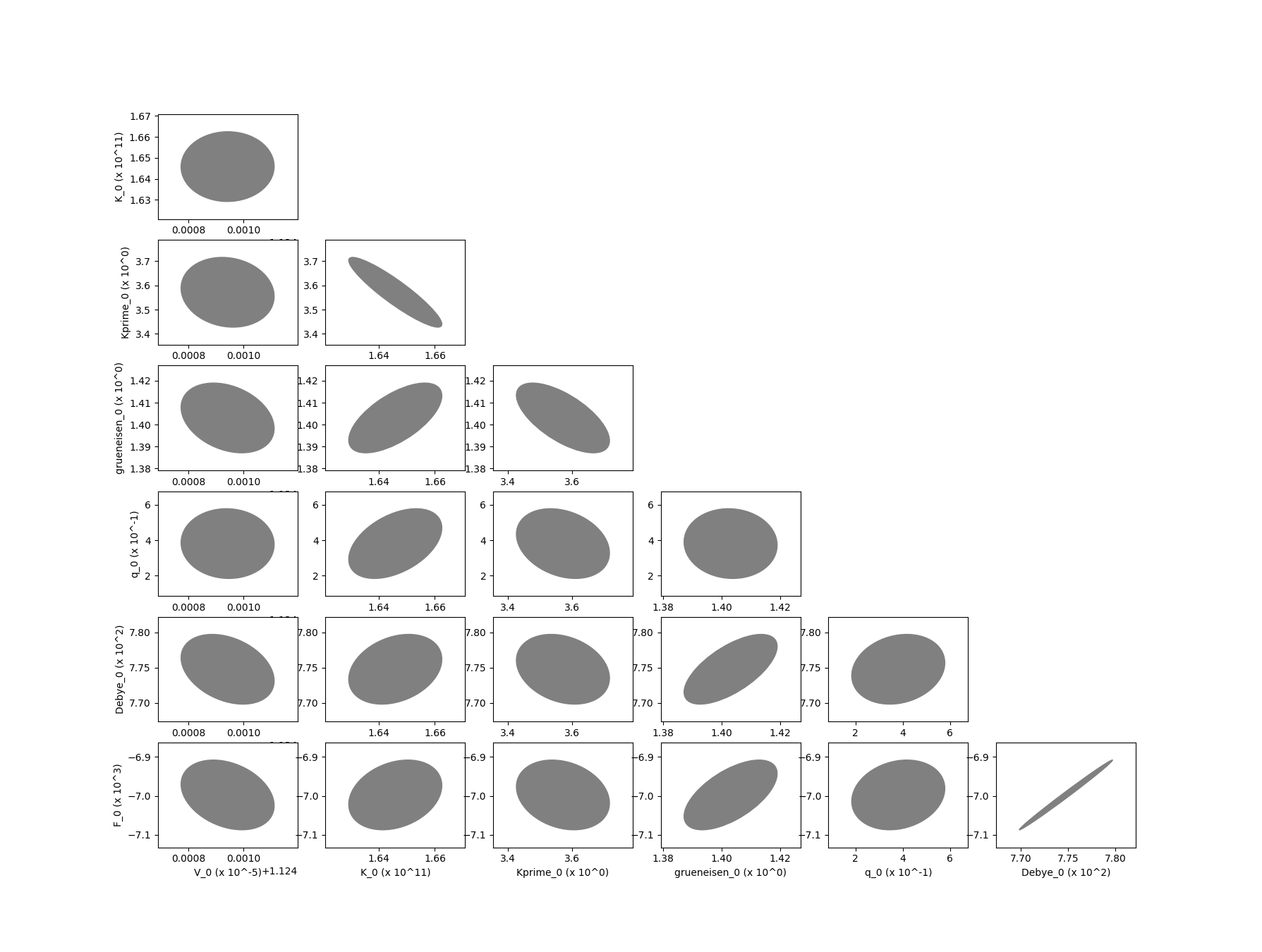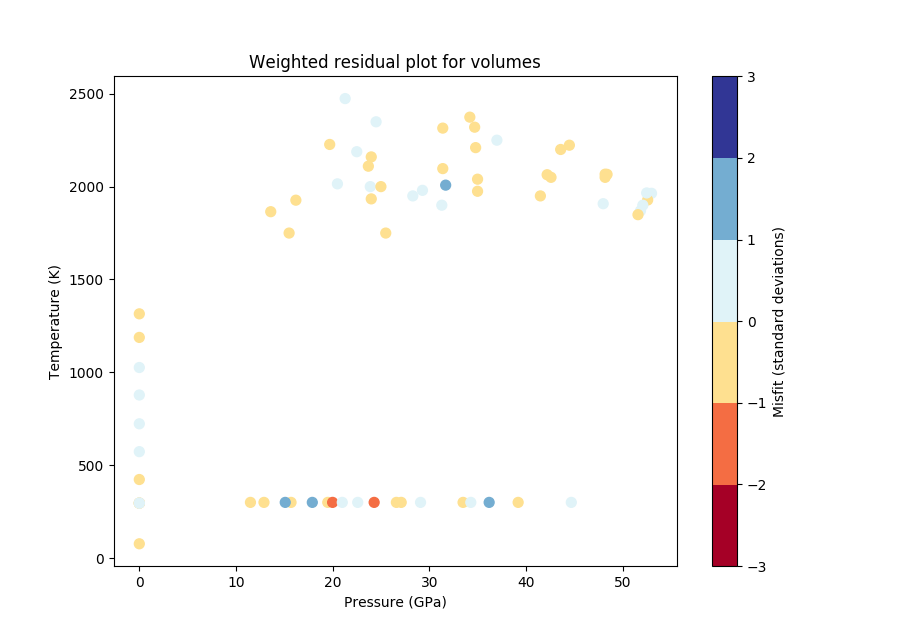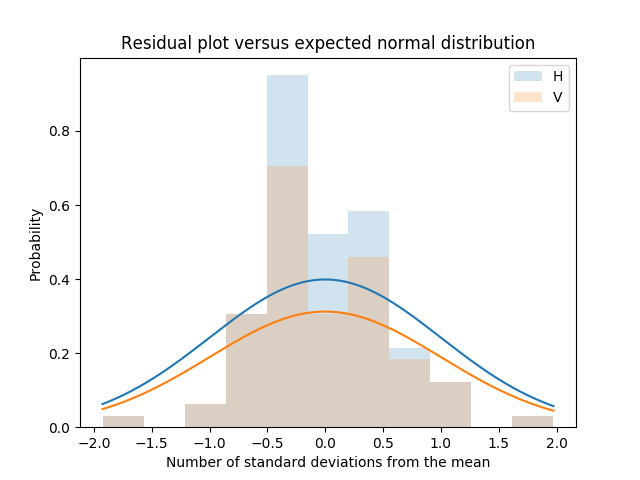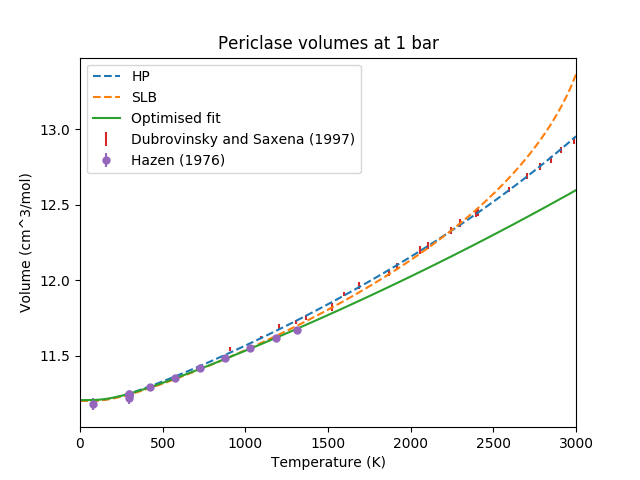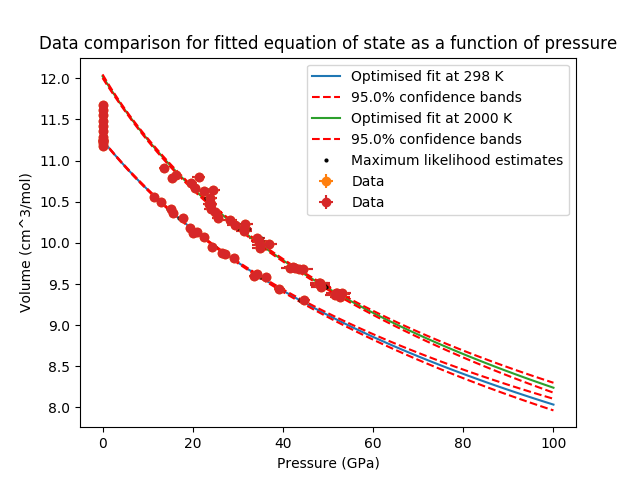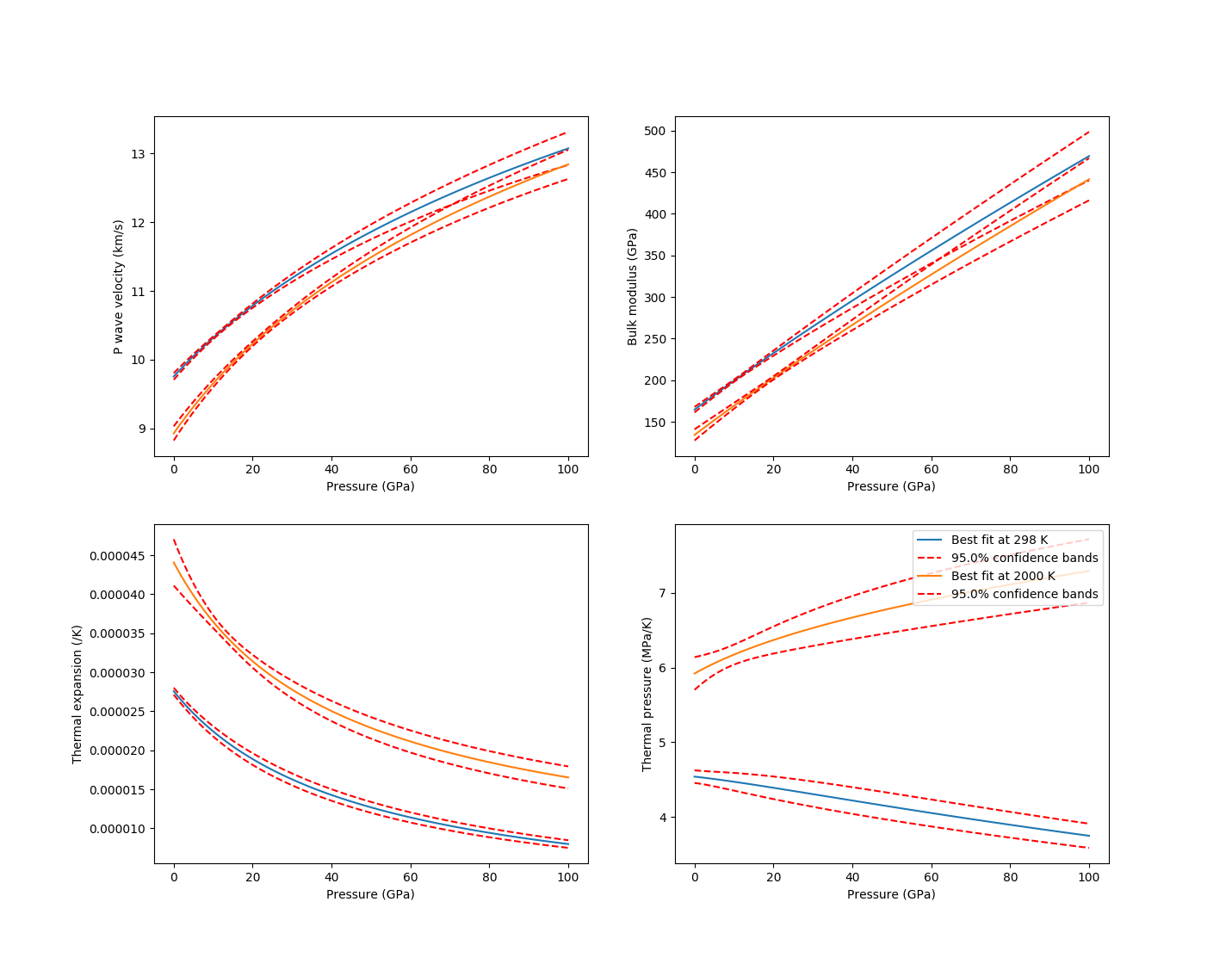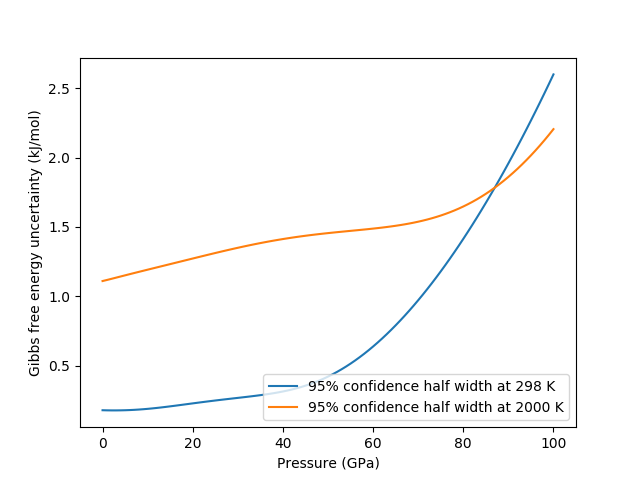## Reproducing Cottaar, Heister, Rose and Unterborn (2014)¶

In this section we include the scripts that were used for all computations and figures in the 2014 BurnMan paper: Cottaar, Heister, Rose & Unterborn (2014) [CHRU14]

### paper_averaging¶

This script reproduces [CHRU14], Figure 2.

This example shows the effect of different averaging schemes. Currently four averaging schemes are available: 1. Voight-Reuss-Hill 2. Voight averaging 3. Reuss averaging 4. Hashin-Shtrikman averaging

See [WDOConnell76] for explanations of each averaging scheme.

requires: - geotherms - compute seismic velocities

teaches: - averaging

### paper_incorrect_averaging¶

This script reproduces [CHRU14], Figure 5. Attempt to reproduce Figure 6.12 from [Mur13]

### paper_opt_pv¶

This script reproduces [CHRU14], Figure 6. Vary the amount perovskite vs. ferropericlase and compute the error in the seismic data against PREM.

requires: - creating minerals - compute seismic velocities - geotherms - seismic models - seismic comparison

teaches: - compare errors between models - loops over models

### paper_onefit¶

This script reproduces [CHRU14], Figure 7. It shows an example for a best fit for a pyrolitic model within mineralogical error bars.

### paper_uncertain¶

This script reproduces [CHRU14], Figure 8. It shows the sensitivity of the velocities to various mineralogical parameters.

## Misc or work in progress¶

### example_grid¶

This example shows how to evaluate seismic quantities on a $$P,T$$ grid.

### example_woutput¶

This example explains how to perform the basic i/o of BurnMan. A method of calculation is chosen, a composite mineral/material (see example_composition.py for explanation of this process) is created in the class “rock,” finally a geotherm is created and seismic velocities calculated.

Post-calculation, the results are written to a simple text file to plot/manipulate at the user’s whim.

requires: - creating minerals - compute seismic velocities - geotherms

teaches: - output computed seismic data to file